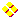WON plate27 | World!OfNumbersPattern excerpts from Mathematical Recreations books From “Mathematical Recreations & Essays” by W.W.Rouse Ball & H.S.M. Coxeter, Twelfth Ed. p. 13,14. Find all numbers which are integral multiples of their reversals. Answer (Sloane's A031877) : for instance, among numbers of four digits, 8712 = 4 x 2178 and 9801 = 9 x 1089 Numbers that are integer multiples of their reversals are called palintiples. Dan Hoey made a study and published his Solution to the /arithmetic/digits/palintiples problem.From “Mathematical Magic Show” by Martin Gardner, page 211 Any number of  9's can be inserted in the middle of each number to obtain larger (but dull) numbers with the same property; for instance, 21999978 x 4 = 87999912. Larger numbers can also be fabricated by repeating each fourdigit number: thus, 2178 2178 2178 x 4 = 8712 8712 8712 and 1089 1089 1089 x 9 = 9801 9801 9801. Of course numbers such as 21999978 may also be repeated to produce reversible numbers. Some considerations 1089 is the square of a palindrome namely ( 33 ) 9801 is the square of a palindrome namely ( 99 ) 9801 – 1089 equals 8712 which is the first example ! 8712 – 2178 equals 6534 6534 – 4356 equals 2178. The circle is closed !From “Figuring - The Joy of Numbers” by Shakuntala Devi, page 70 and 122 "Numbers made up only of threes have a special pattern of squares" 33 2 = 1089 333 2 = 110889 3333 2 = 11108889 33333 2 = 1111088889 333333 2 = 111110888889 Note that 33 equals 1! + 2! + 3! + 4! and that 33 equals 14 + 25 "The number 1089 has some peculiar traits. For instance look at the pattern that is formed when it is multiplied by the numbers 1 to 9 :" 1089 x 1 = 1089 --- 9801 = 1089 x 9 1089 x 2 = 2178 --- 8712 = 1089 x 8 1089 x 3 = 3267 --- 7623 = 1089 x 7 1089 x 4 = 4356 --- 6534 = 1089 x 6 1089 x 5 = 5445The following comes from Shakuntala Devi's book “Figuring - The Joy of Numbers” page 123 : Multiplying the number 12345679 by the multiples of 9, gives a curious set of palindromic patterns : 12345679 x 9 = 111111111 12345679 x 18 = 222222222 12345679 x 27 = 333333333 and so on. Multiplying by 999999999 itself gives a number which is a mirror image of itself 12345679 x 999999999 = 12345678987654321 incidentally, this product is a perfect square. It's also Palindromic Square  111111111 2 = 12345678987654321 Many people find the above square quite useless but nevertheless fascinating !A000027Prime Curios!Prime PuzzleRichard PhillipsWikipedia 27Le Nombre 27Numberland 27```

```

[TOP OF PAGE]

Patrick De Geest - Belgium- Short Bio - Some Pictures